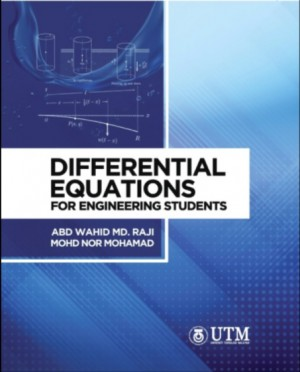## Differential Equations for Engineering Students

RM 46.00

### ISBN:

978-983-52-1743-2

99.66 MB

epub

English

2021
Favorite (6)

### Author

Abd Wahid Md Raji, Mohd Nor Mohamad

### Publisher

Penerbit UTM Press

### Synopsis

This book consists of seven chapters. Since ordinary differential equations are probably the most immediately useful part of calculus and also the techniques for solving simple ordinary differential equations stem naturally from calculus, therefore it is wise that this book begins with a chapter on the basic concept of ordinary differential equations. The next chapters that follow are the first and second order of ordinary differential equations with constant coefficients and their applications, higher order equations, Laplace transforms, Fourier series and an introduction to partial differential equations. Each chapter has been carefully planned to enable students integrate the underlying theory, solution procedures, and computational aspects as seamlessly as possible